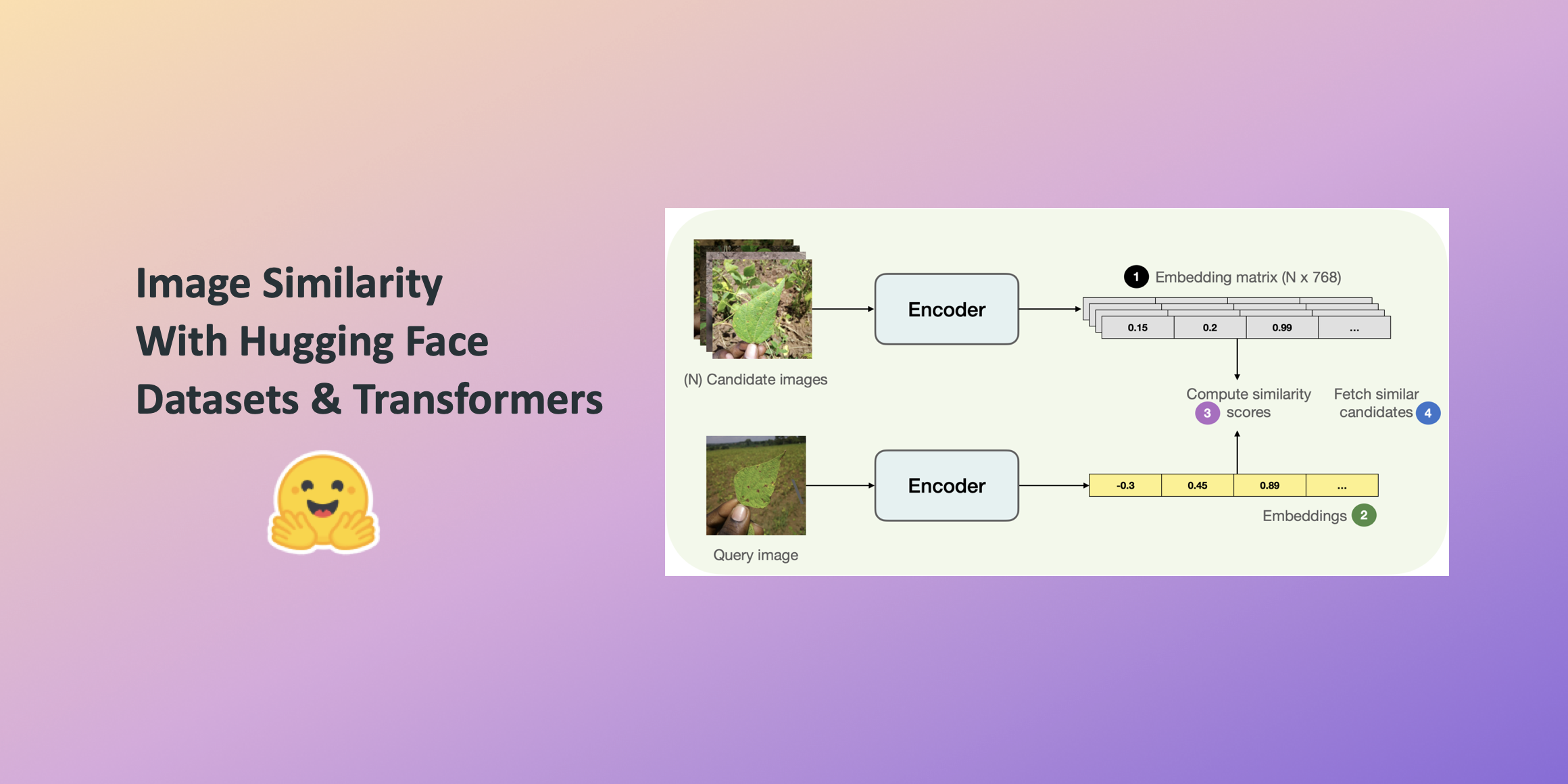## Highlights §

• To build this system, we first need to define how we want to compute the similarity between two images. One widely popular practice is to compute dense representations (embeddings) of the given images and then use the cosine similarity metric to determine how similar the two images are. (View Highlight)
• e’ll use “embeddings” to represent images in vector space. This gives us a nice way to meaningfully compress the high-dimensional pixel space of images (224 x 224 x 3, for example) to something much lower dimensional (768, for example). The primary advantage of doing this is the reduced computation time in the subsequent steps. (View Highlight)
• To compute the embeddings from the images, we’ll use a vision model that has some understanding of how to represent the input images in the vector space. This type of model is also commonly referred to as image encoder. (View Highlight)
• instead of using a generalist model (like the ones trained on the ImageNet-1k dataset, for example), it’s better to use a model that has been fine-tuned on the dataset being used. That way, the underlying model better understands the input images. Note that you can also use a checkpoint that was obtained through self-supervised pre-training. The checkpoint doesn’t necessarily have to come from supervised learning. In fact, if pre-trained well, self-supervised models can yield impressive retrieval performance. Now that we have a model for computing the embeddings, we need some candidate images to query against. (View Highlight)
• he process of finding similar images Below, you can find a pictorial overview of the process underlying fetching similar images.(View Highlight)
• • Extract the embeddings from the candidate images (candidate_subset), storing them in a matrix. • Take a query image and extract its embeddings. • Iterate over the embedding matrix (computed in step 1) and compute the similarity score between the query embedding and the current candidate embeddings. We usually maintain a dictionary-like mapping maintaining a correspondence between some identifier of the candidate image and the similarity scores. • Sort the mapping structure w.r.t the similarity scores and return the underlying identifiers. We use these identifiers to fetch the candidate samples. (View Highlight)
• We can write a simple utility and map() it to our dataset of candidate images to compute the embeddings efficiently. import torch def extract_embeddings(model: torch.nn.Module): """Utility to compute embeddings.""" device = model.device def pp(batch): images = batch[“image”]

# for the model. For more details, check out the accompanying Colab Notebook. §

image_batch_transformed = torch.stack( [transformation_chain(image) for image in images] ) new_batch = {“pixel_values”: image_batch_transformed.to(device)} with torch.no_grad(): embeddings = model(**new_batch).last_hidden_state[:, 0].cpu() return {“embeddings”: embeddings} return pp And we can map extract_embeddings() like so: device = “cuda” if torch.cuda.is_available() else “cpu” extract_fn = extract_embeddings(model.to(device)) candidate_subset_emb = candidate_subset.map(extract_fn, batched=True, batch_size=batch_size) (View Highlight)
• ext, for convenience, we create a list containing the identifiers of the candidate images. candidate_ids = [] for id in tqdm(range(len(candidate_subset_emb))): label = candidate_subset_emb[id][“labels”]

# Create a unique indentifier. §

entry = str(id) + ”_” + str(label) candidate_ids.append(entry) We’ll use the matrix of the embeddings of all the candidate images for computing the similarity scores with a query image. We have already computed the candidate image embeddings. In the next cell, we just gather them together in a matrix. (View Highlight)
• all_candidate_embeddings = np.array(candidate_subset_emb[“embeddings”]) all_candidate_embeddings = torch.from_numpy(all_candidate_embeddings) We’ll use cosine similarity to compute the similarity score in between two embedding vectors. We’ll then use it to fetch similar candidate samples given a query sample. def compute_scores(emb_one, emb_two): """Computes cosine similarity between two vectors.""" scores = torch.nn.functional.cosine_similarity(emb_one, emb_two) return scores.numpy().tolist() def fetch_similar(image, top_k=5): """Fetches the top_k similar images with image as the query."""

# Prepare the input query image for embedding computation. §

image_transformed = transformation_chain(image).unsqueeze(0) new_batch = {“pixel_values”: image_transformed.to(device)}

# Comute the embedding. §

with torch.no_grad(): query_embeddings = model(**new_batch).last_hidden_state[:, 0].cpu()

# and their similarity scores with the query image. §

sim_scores = compute_scores(all_candidate_embeddings, query_embeddings) similarity_mapping = dict(zip(candidate_ids, sim_scores))

# Sort the mapping dictionary and return top_k candidates. §

similarity_mapping_sorted = dict( sorted(similarity_mapping.items(), key=lambda x: x, reverse=True) ) id_entries = list(similarity_mapping_sorted.keys())[:top_k] ids = list(map(lambda x: int(x.split(””)), id_entries)) labels = list(map(lambda x: int(x.split(””)[-1]), id_entries)) return ids, labels (View Highlight)
• • If we store the embeddings as is, the memory requirements can shoot up quickly, especially when dealing with millions of candidate images. The embeddings are 768-d in our case, which can still be relatively high in the large-scale regime. • Having high-dimensional embeddings have a direct effect on the subsequent computations involved in the retrieval part. (View Highlight)
• If we can somehow reduce the dimensionality of the embeddings without disturbing their meaning, we can still maintain a good trade-off between speed and retrieval quality. The accompanying Colab Notebook of this post implements and demonstrates utilities for achieving this with random projection and locality-sensitive hashing. (View Highlight)